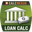# Loan Calculator

Leave the field blank that you wish to solve for.
 Loan Amount x Annual % Rate x Term (years) x Payment x Frequency MonthlySemi-MonthlyBi-WeeklyWeeklyBi-MonthlyQuarterlySemi-AnnuallyAnnually

## DescriptionThis simple loan calculator lets you solve for the Principal, Rate, Term, or Payment for a fixed-rate loan or mortgage. The calculator estimates the Total Interest paid over the course of the loan.

The payment includes Principal+Interest, but not Insurance and Property taxes. It does not take into account rounding, so the estimate may be off be a few dollars. Note also that the "bi-weekly" payment option is not the same as "accelerated bi-weekly" payments, in which an extra payment is made.

Solving for the Rate requires iteration, and there may be zero or more solutions. When the solver fails to converge, you'll get an error such as "Could not solve for Rate". The rate is rounded to the 6th decimal place.

Extra Payments: To estimate the effect of making extra payments, follow these 2 steps ...
Step 1: Calculate the normal payment. For example, a 150,000 loan at 5% for 30 yrs = \$805.23 per month. Write down the total interest, which is about \$139,884
Step 2: Clear the Term field and add \$100 to the monthly payment so that the Payment field is \$905.23. Press Calc and you'll see that the loan is paid off after 23.5 years (the calculated Term) and the total interest is about \$105,279, saving you about \$34,600 in interest. In this calculator, we have defined the "Term" as the number of years to pay off the loan.

Loan Amount - This is the amount that you have borrowed.

Annual Rate % - This is the annual interest rate quoted by the lender.

Term of Loan - The total number of years it will take to pay off the loan.

Payment - This is the PI (Principal + Interest) amount that you'll pay each period.

Payment Frequency - Used to specify the number of payments made per year (Monthly = 12 payments per year, Semi-Monthly = 24 per year, Bi-Weekly = 26 per year).

Note: Values returned by this calculator may not be exact, due to rounding or truncation errors. Also, this calculator assumes the compounding period is the same as the payment frequency.

Disclaimer: Your financial situation is unique, and circumstances vary, so don't depend on this Mortgage calculator to make your home financing decisions. Please consult a professional. This calculator is for informational use only and does not constitute tax or financial advice.

## More Loan Calculators

If you use Excel, try our Simple Loan Calculator for Excel